### Sample Problem

Look at the figure below. If the area of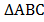is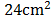, AD = DB, CE = 2 BE, then what is the area of?

cm2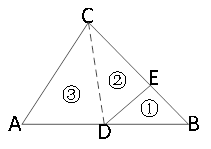#### Solution

The key to this problem is to find the relation between the area ofand the area of. We draw line segment CD to create triangles with same heights. Sinceandshare a same height, but CE is 2 times BE, thushas an area two times that of. Since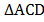and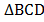share a same height, and AD = DB, thus the areas of these two triangles are the same. If we call the area of, (1) , thenis 2 (1) , and= (1) + 2 (1) = 3 (1) .

Thus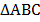= (1) + 2 (1) + 3 (1) = 6 (1) .

We already know this equals 24. So we have

6 (1) = 24, thus (1) = 24 cm2 ÷ 6 = 4 cm2